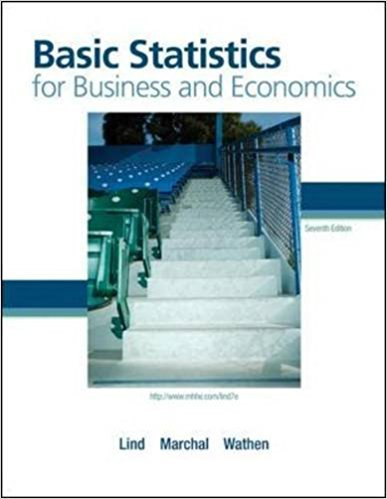×
Get Full Access to Basic Statistics For Business And Economics - 7 Edition - Chapter 5 - Problem 2e
Get Full Access to Basic Statistics For Business And Economics - 7 Edition - Chapter 5 - Problem 2e

×

# A quality control inspector selects a part to be tested.ISBN: 9780077384470 64

## Solution for problem 2E Chapter 5

Basic Statistics for Business and Economics | 7th Edition

• Textbook Solutions
• 2901 Step-by-step solutions solved by professors and subject experts
• Get 24/7 help from StudySoup virtual teaching assistantsBasic Statistics for Business and Economics | 7th Edition

4 5 1 294 Reviews
12
1
Problem 2E

Problem 2E

A quality control inspector selects a part to be tested. The part is then declared acceptable, repairable, or scrapped. Then another part is tested. List the possible outcomes of this experiment regarding two parts.

Step-by-Step Solution:

Step 1 of 1:

(a)

We are asked to list the possible outcomes of this experiment regarding two parts.

A quality control inspector selects a part to be tested.

The part is then declared acceptable, repairable, or scrapped.

Since the number of parts is selected is two, hence the number of possible outcomes would beLetrepresent the part which is acceptable.

Letrepresent the part which is repairable.

Hence the possible outcomes are,

 Part Outcome 1 2 1 A A 2 A R 3 R A 4 R R

Step 2 of 1

## Discover and learn what students are asking

Calculus: Early Transcendental Functions : The Natural Logarithmic Function: Integration
?In Exercises 1-26, find the indefinite integral. $$\int \frac{10}{x} d x$$

Calculus: Early Transcendental Functions : Area and Arc Length in Polar Coordinates
?In Exercises 5-16, find the area of the region. One petal of $$r=\sin 2 \theta$$

Statistics: Informed Decisions Using Data : Comparing Three or More Means (One-Way Analysis of Variance)
?The variability within each treatment group, which is a weighted average of the sample variances from each treatment where the weights are based on th

Unlock Textbook Solution